# Work and Energy - NCERT Questions

Q 1.

A force of 7 N acts on an object. The displacement is, say 8 m, in the direction of the force as shown in figure. Let us take it that the force acts on the object through the displacement. What is the work done in this case?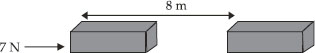SOLUTION:

Force, F = 7 N
Displacement, s = 8 m
Then, Work done = 7 N × 8 m
= 56 N m = 56 J

Q 2.

When do we say that work is done?

SOLUTION:

Work is done by a force on an object if a force acts on the object and the object is displaced from its original position.

Q 3.

Write an expression for the work done when a force is acting on an object in the direction of its displacement.

SOLUTION:

If F is the constant force acting in the direction of displacement s, then work done by the force, i.e., W = F × s = Fs.

Q 4.

Define 1 J of work.

SOLUTION:

The amount of work done when a force of 1 N moves a body through a distance of 1 m in the direction of the force is called 1 joule.

Q 5.

A pair of bullocks exerts a force of 140 N on a plough. The field being ploughed is 15 m long. How much work is done in ploughing the length of the field?

SOLUTION:

Here, F = 140 N, s = 15 m.
Work done in ploughing the field,
W = F × s = (140 N) × (15 cm) = 2100 J

Q 6.

What is the kinetic energy of an object?

SOLUTION:

Kinetic energy of an object is the energy possessed by it due to its motion. In fact, kinetic energy of an object moving with a certain velocity is equal to the work done to make it acquire that velocity.

Q 7.

Write an expression for the kinetic energy of an object.

SOLUTION:

Kinetic energy of an object,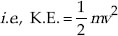where m = mass of the object and
v = uniform velocity of the object

Q 8.

The kinetic energy of an object of mass, m moving with a velocity of 5 m s–1 is 25 J. What will be its kinetic energy when its velocity is doubled? What will be its kinetic energy when its velocity increased three times?

SOLUTION:

K.E. of an object = 25 J
K.E. of the object with velocity double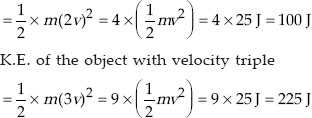Q 9.

What is power?

SOLUTION:

The rate of doing work is called power.
Thus,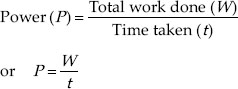Power may also be defined as the amount of work done in one unit of time.

Q 10.

Define 1 watt of power.

SOLUTION:

Power of an object is said to be 1 W if it does 1 joule of work in 1 s, i.e.,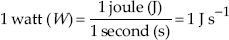In other words, we say that power is 1 W when the rate of consumption of energy is 1 J s–1.

Q 11.

A lamp consumes 1000 J of electrical energy in 10 s. What is its power?

SOLUTION:

Energy consumed = 1000 J
So, Time = 10 s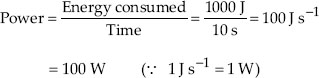Q 12.

Define average power.

SOLUTION: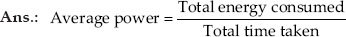The concept of average power is useful when the power of an agent varies with time and it does work at different rates during different intervals of time.

Q 13.

Look at the activities listed below. Reason out whether or not work is done in the light of your understanding of the term ‘work’.
• Suma is swimming in a pond.
• A donkey is carrying a load on its back.
• A wind-mill is lifting water from a well.
• A green plant is carrying out photosynthesis.
• An engine is pulling a train.
• Food grains are getting dried in the sun.
• A sailboat is moving due to wind energy.

SOLUTION:

(A) Suma is doing work. She is applying force to move horizontally.
(B) Donkey is not doing any work. Here, the displacement and the force are at 90°.
(C) Work is done by the windmill. The water is lifted against force of gravity.
(D) No work is done by a green plant during photosynthesis.
(e) The engine applies a pulling force on the train, and the train moves in the direction of this force. Therefore, engine is doing work.
(f) During drying of food grains in the sun no work is done.
(g) Work is done by the air. The sailboat moves in the direction of the force exerted by wind.

Q 14.

An object thrown at a certain angle to the ground moves in a curved path and falls back to the ground. The initial and the final points of the path of the object lie on the same horizontal line. What is the work done by the force of gravity on the object?

SOLUTION:

Since initial and the final positions of the path of the object thrown at a certain angle to the ground lie on the some horizontal plane, the displacement of the object is in the horizontal direction. The force of gravity on the object acts vertically downwards, so no work is said to be done.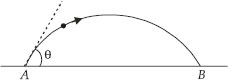Q 15.

A battery lights a bulb. Describe the energy changes involved in the process.

SOLUTION:

A battery converts chemical energy into electrical energy. This electrical energy is converted into light energy as the bulb is lighted up, i.e., the sequence of energy changes is as follows :
Chemical energy → Electrical energy → Light energy.

Q 16.

Certain force acting on a 20 kg mass changes its velocity from 5 m s–1 to 2 m s–1. Calculate the work done by the force.

SOLUTION:

Mass of the object, m = 20 kg
Total work done by the force = Change in the kinetic energy of the object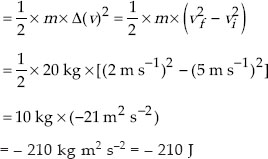The force will do work equivalent to 210 J. Here the direction of force is opposite to the direction of motion.

Q 17.

A mass of 10 kg is at a point A on a table. It is moved to a point B. If the line joining A and B is horizontal, what is the work done on the object by the gravitational force? Explain your answer.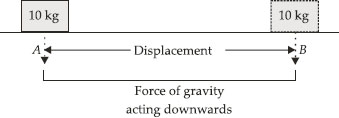SOLUTION:

Here, the mass of 10 kg is moved horizontally from point A to B. The force of gravity acts vertically downwards. Thus, the mass moves in a direction at right angle to the force of gravity. So,
Work done on the object by the force of gravity = FScosθ = FScos90° = 0
Therefore, no work is done by the force of gravity on the object.

Q 18.

The potential energy of a freely falling object decreases progressively. Does this violate the law of conservation of energy? Why?

SOLUTION:

As the potential energy of the freely falling object decreases, its kinetic energy increases on account of an increase in its velocity. The sum total of the potential energy and the kinetic energy of the object during its free fall remains the same, i.e., the total mechanical energy (potential energy + kinetic energy) remains constant. Thus, the law of conservation of energy is not violated.

Q 19.

What are the various energy transformations that occur when you are riding a bicycle?

SOLUTION:

The muscular energy of the cyclist is converted into kinetic energy (rotational) of the pedals of the bicycle which is transferred to its wheels. The kinetic energy of the rotation of the wheels is converted into the kinetic energy of the bicycle and the cyclist.

Q 20.

Does the transfer of energy take place when you push a huge rock with all your might and fail to move it? Where is the energy you spend going?

SOLUTION:

No transfer of energy takes place when we push a huge rock unsuccessfully.
The energy is spent for the physical activity of muscles.

Q 21.

A certain household has consumed 250 units of energy during a month. How much energy is this in joules?

SOLUTION:

Energy consumed = 250 units = 250 kW h
= 250 × 1000 W h = 250 × (1000 W) (3600 s)
= 250 × 1000 × 3600 W s
= 900 × 106 = 9 × 108 J(∵ 1 W = 1 J s–1)

Q 22.

An object of mass 40 kg is raised to a height of 5 m above the ground. What is its potential energy? If the object is allowed to fall, find its kinetic energy when it is half-way down.

SOLUTION:

Potential energy of the object = m × g × h
= 40 kg × 10 m s–2 × 5 m
= 40 × 10 × 5 kg m s–2 m = 2000 J
Let v be the velocity of the object at half the height i.e., after travelling 2.5 m.
Then v2u2 = 2as
v2 – 02 = 2 × 10 m s–2 × 2.5 m = 50 m2 s–2
So, v2 = 50 m2 s–2
Then,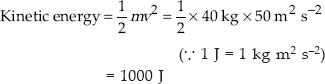Q 23.

What is the work done by the force of gravity on a satellite moving round the earth? Justify your answer.

SOLUTION:

When a satellite moves around the earth, its displacement in a short interval is along the tangent to the circular path of the satellite. The gravitational force (F) acting on the satellite due to the earth is along the radius as shown in figure.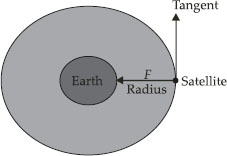Since a tangent is always perpendicular to the radius, the displacement and the force are perpendicular to each other. There is no displacement of the satellite in the direction of the force, i.e., s = 0. Thus, work done by the force of gravity on the satellite is zero as W = F × s = 0.

Q 24.

Can there be displacement of an object in the absence of any force acting on it? Think. Discuss this question with your friends and teacher.

SOLUTION:

Yes. In the absence of any force on the object, i.e. F = 0, ma = 0 (as F = ma). Since
m ≠ 0, a = 0. In such a case, the object is either at rest or in a state of uniform motion in a straight line. In the latter case, there is a displacement of the object without any force acting on it.

Q 25.

A person holds a bundle of hay over his head for 30 minutes and gets tired. Has he done some work or not? Justify your answer.

SOLUTION:

The person holding a bundle of hay on his head gets tired as he experiences muscular fatigue. This fatigue is due to the conversion of chemical energy into thermal energy by the muscular effort. The person has done no work as his effort causes no displacement of the bundle over his head, i.e., s = 0.

Q 26.

An electric heater is rated 1500 W. How much energy does it use in 10 hours?

SOLUTION:

Here, power of the electric heater, P = 1500 W
Time for which it is used, t = 10 h
Energy used by the electric heater, i.e.,
W = P × t = 1500 W × 10 h = 15000 W h
= 15 kW h = 15 units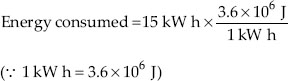Q 27.

Illustrate the law of conservation of energy by discussing the energy changes which occur when we draw a pendulum bob to one side and allow it to oscillate. Why does the bob eventually come to rest? What happens to its energy eventually? Is it a violation of the law of conservation of energy?

SOLUTION:

A small metallic ball (called bob) suspended by a light string (thread) from a frictionless, rigid support is called a simple pendulum. A simple pendulum is shown in figure alongside.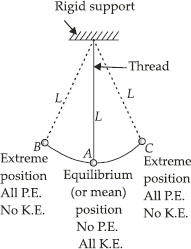The bob of a simple pendulum swings from one extreme position to the other through its mean position.
The bob is at its highest position at the extreme positions and at lowest at its mean position.
The energy changes which occur during the motion of the bob are shown in figure. So, when a pendulum swings to and fro, its energy changes constantly in the following sequence.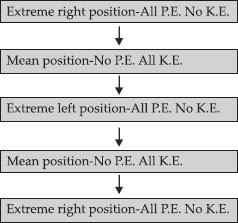Q 28.

An object of mass, m is moving with a constant velocity, v. How much work should be done on the object in order to bring the object to rest?

SOLUTION:

Initial kinetic energy of the object,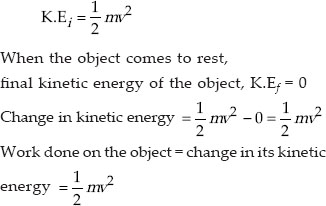Q 29.

Calculate the work required to be done to stop a car of 1500 kg moving at a velocity of 60 km h–1?

SOLUTION:

Here, mass of the car, m = 1500 kg
Initial velocity of the car, u = 60 km h–1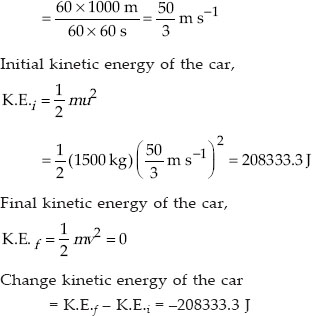The sign of minus indicates that the work is being done against the moving car.

Q 30.

In each of the following a force, F is acting on an object of mass, m. The direction of displacement is from west to east shown by the longer arrow. Observe the diagrams carefully and state whether the work done by the force is negative, positive or zero.
(A)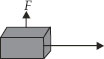(B)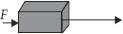(C)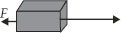SOLUTION:

(A)Here, the force acts at right angle to the displacement. So,
work done by the force = 0
(B) Here, the direction of displacement is the same as that of the force. So,
work done by the force = + ve
(C) Here, the body moves in a direction opposite to the direction of the force. So,
work done by the force = – ve

Q 31.

A force of 7 N acts on an object. The displacement is, say 8 m, in the direction of the force as shown in figure. Let us take it that the force acts on the object through the displacement. What is the work done in this case?SOLUTION:

The acceleration of an object can be zero even if several forces are acting on it provided the resultant force (F) is zero. As F = 0, ma = 0 and accordingly a = 0 (as m ≠ 0).

Q 32.

Find the energy in kW h consumed in 10 hours by four devices of power 500 W each.

SOLUTION:

Power of each device = 500 W
Number of devices = 4
Duration of use = 10 h
Total energy consumed = 4 × 500 W × 10 h
= 20000 Wh
= 20 kW h

Q 33.

A freely falling object eventually stops on reaching the ground. What happens to its kinetic energy?

SOLUTION:

When a freely falling body eventually stops on reaching the ground, its kinetic energy appears in the form of :
(i) heat (the body and the ground become warmer due to collision).
(ii) sound (produced due to collision of the body with the ground).
(iii) potential energy of configuration of the body and the ground (the body may be deformed and the ground may be depressed at the place of collision).
This process in which the kinetic energy of a freely falling body is lost in an unproductive chain of energy changes is called dissipation of energy.